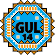General Utility Library for C++14  2.9
gul14::expected< T, E > Class Template Reference

## Detailed Description

### template<typename T, typename E> class gul14::expected< T, E >

An `expected<T, E>` is an object that normally contains an "expected" object of type T, but it can alternatively contain another, "unexpected" or "error" object of type E.

The main use case of `expected` is as a function return value. It is a more expressive alternative to the common idiom of returning a `bool` to indicate success or failure, and using an output parameter to return the result:

std::expected<double, std::string> square_root(double v)
{
if (v < 0.0)
return std::unexpected<std::string>("negative value");
else
return std::sqrt(v);
}
for (double v = -2.0; v <= 2.0; v += 0.5)
{
auto result = square_root(v);
if (result)
std::cout << result.value() << "\n";
else
std::cout << result.error() << "\n";
}
Note
The GUL14 version is an adaptation of Sy Brand's implementation (see expected.h) and should behave like std::expected from C++23 for most use cases.
Since
GUL version 2.8.0

The documentation for this class was generated from the following file: